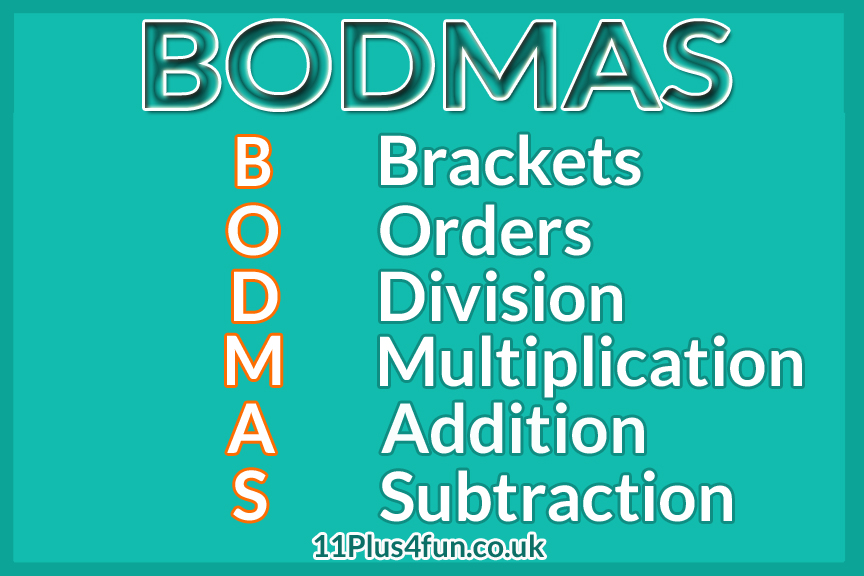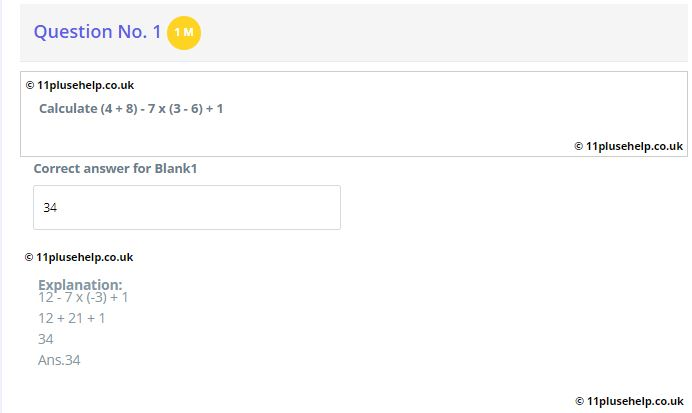# 11 Plus BODMAS

11 Plus BODMAS

We have plenty of 11 Plus Practice Tests on BODMAS at 11PluseHelp.co.uk

Example 1

Can you tell the answer of ( 5 + 6 ) × 4?

( 5 + 6 ) × 4

First Step
In the first step, we should solve the bracket according to the BODMAS rule.

After solving the bracket, we get the answer 11.

11 x 4

Second Step
Now, we have to multiply 11 and 4. After multiplication, we get the answer 44.

44

We all agree that Mathematics has become the basic need of human being. That’s why we all must learn at least basic mathematical concepts. In mathematics, we have to solve lots of problems. Some problems are easy for us to solve, while some complicated problems are hard to solve.

With a simple sum with two numbers and one single operation, or sign, it’s easy to calculate the answer. It may be adding, subtracting, multiplying, or dividing. It means that if we are going to solve mathematical problems like (12 x 8), (16/2), (6 – 5), and so on, it is easy for us to solve these kinds of problems because these kinds of problems have only one operation.

On the other hand, when there are several numbers, and different operations, then how to solve the problem? Maybe you need to divide and multiply, or add and divide. What do you do then? When we are going to solve some arithmetic operations that involve more than one operation like (12×4-3+2), it is hard for us to understand how to solve this problem.

Fortunately, mathematics is a logic-based discipline. There are some simple rules to follow that help you work out the order to do the sum. This rule is called as BODMAS rule. This rule was introduced by Achilles Reselfelt as early as 1600. The main aim of introducing this kind of mathematical rule was to solve those problems which involve more than one mathematical operation in a mathematical problem.

BODMAS is an acronym. Using this specific rule makes it easy for us to keep in mind the order of operations while solving different kinds of mathematical problems or operations.

The meanings of different words of this acronym are given below;1. B — Bracket

It means that first of all, we have to solve the brackets in the mathematical operation.

There are four different kinds of brackets.

First of all, we have to solve “━━━━”.

Secondly, we have to solve ().

Thirdly, we have to solve {}.

At last, we have to solve [].

1. O — Of or Orders

It means that you will have to solve all the numbers which have powers and brackets.

1. D — Division

It means that you have to perform the division operation.

1. M — Multiplication

After that, you have to perform the multiplication operation.

After that, you have to add the numbers or terms.

1. S — Subtraction

At last, you have to subtract the numbers and terms.

11 Plus Topic-wise Free Practice Papers:

11 Plus Topic-wise Free Practice Papers

Free 11 Plus BODMAS Practice Papers:

Free 11 Plus BODMAS Practice Papers

Let’s see some examples of usage of the BODMAS rule:

### Example 1

4[2 + { 7(5-3)}]

#### First Step

In the first step, we have to solve (). After solving it, we get:

4 [ 2 + { 7 x 2 } ]

#### Second Step

Now, we have to solve { }. After solving it, we get:

4 [ 2 + 14 ]

#### Third Step

Now, we have to solve [ ]. After solving it, we get:

4 x16

At last, we have to multiply these two terms. After multiplying 4 and 16, we get the answer 64.

64

### Example 2

20 x 2 – ( 4 / 2 ) x 9 x 2^2

#### First Step

20 x 2 – ( 4 / 2 ) x 9 x 2^2

#### Second Step

20 x 2 – 2 x 9 x 2^2

#### Third Step

20 x 2 – 2 x 9 x 4

20 x 2 – 72

40 – 72

#### Sixth Step

-32

By following this specific rule, you will be able to solve any mathematical operation easily and perfectly. We have plenty of 11 Plus Practice tests on BODMAS at 11PluseHelp.co.uk11PluseHelp.co.uk is a one-stop-shop for 11 Plus preparation, including 11 Plus Independent schools preparation. You can have unlimited online practice on 11 Plus subjects – Maths, English, Verbal Reasoning and Non-Verbal Reasoning.

11 Plus Maths Topic-wise Free Practice Tests:

11 Plus Maths Topic-Wise Free Practice Tests

We focus on fundamentals, logic, basics and cover most of the syllabus for all 11 Plus exam patterns in the UK. All these tests lead to perfect practice.

11 Plus Significant Figures, Place Value and BODMAS Questions:

11 Plus Significant Figures, Place Value and BODMAS Questions

For example, We cover Maths topic wise questions and basics and how to apply them.
We have various tests, time-based, short, long and various types, including standard mocks.

You can access 11 Plus FREE Papers by visiting the below link:
https://www.11plusehelp.co.uk/11-plus-free-online-papers

You can access 11 Plus FREE Sample Papers by visiting the below link:
https://www.11plusehelp.co.uk/11-plus-sample-papers

11 Plus complete solution features:

https://www.11plusehelp.co.uk/blog/2017/05/01/11-plus-complete-solution/

Practice and Perseverance Over Genius and Talent

Thanks,

11Plusehelp.co.uk